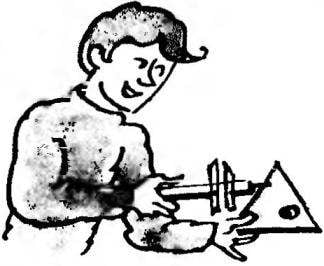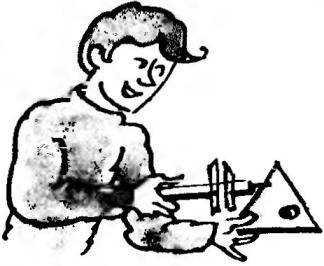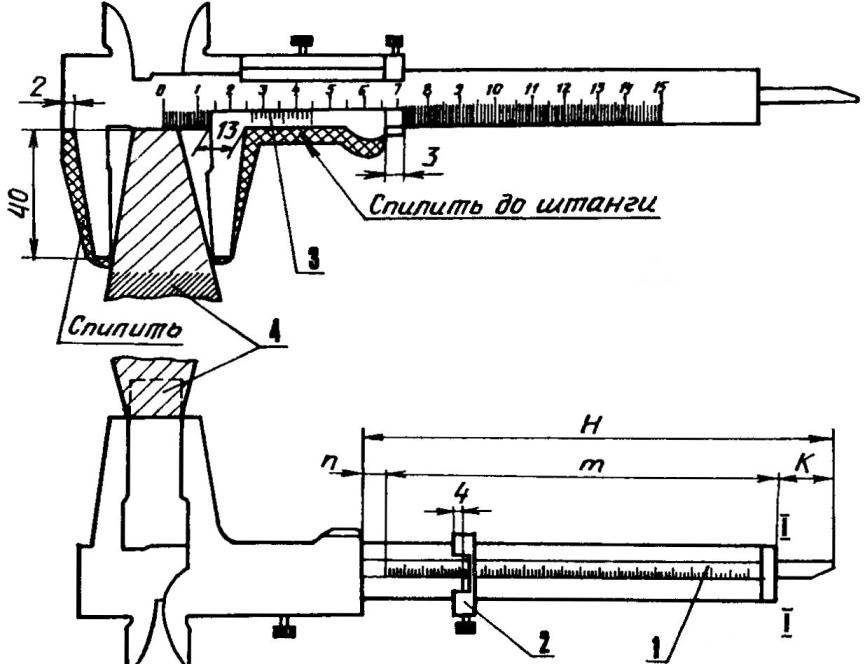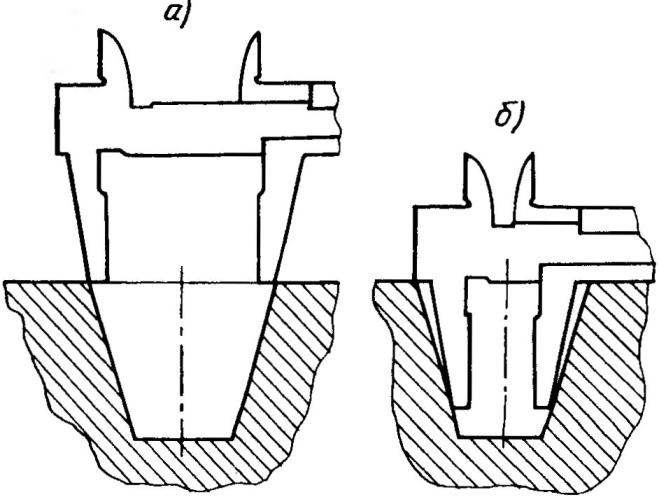# THE CORNER OF THE CALIPERIt turns out that the caliper can be removed not only linear, but also angular dimensions, for example, the amount of taper or slope of different parts. To obtain such a tool, improving, for example, is known to many caliper type SHC-1 (“revolver”) with a probe of the depth gauge. The limit of its linear measurements is 130mm, which will fully satisfy the business needs of Amateur Craftsmen.

The essence of the improvement consists in the following.

Sponge and frame of Vernier it is necessary to prepare according to Fig.1. It does not reduce the rigidity of the tool and will allow you to measure the taper of the external surfaces, and holes and recesses.

The relationship of the measured angles with the length of the jaws is quite simple (Fig. 2): slope angle And the solution of the jaws determined by the formula S = L-tgA, where L is the length of the jaws, equal to 40 mm; taper angle = 2A the solution of the jaws D is determined by the formula-D = 2C = = 2L-tgB/2. Every corner In the corresponding solution of D, that is, if In=1°, D=0.7 mm if=5°, D=3.5 mm if=115°, then D=125,6 mm, etc.
Then the probe of the depth gauge is necessary to provide a scale of angular measurements, the rod is movable with the reticle mark. But before that, it is advisable to determine the distance m corresponding to the measured corner:

m = N — (K+p), where the length of the bar is H = 140 mm, k = D, the distance of the origin p = 4 mm.

So, for an angle of 1° distance m = 140 – (0,7+4) = 135,3 mm 5° – 132,5 mm, for 115° and 10.4 mm, etc.Fig.1. Caliper-inclinometer:

1 —angle measuring scale; 2 — reticle; 3 — frame Vernier; 4 — the body measured.Fig.2. The geometric parameters used in measuring angles.Fig.Z. The position of the jaws of the Vernier caliper when measuring the taper of the hole:

a — first measurement; b — second measurement.

Strokes and numbers on the dipstick it is best to apply a method of etching or embossing, spot, razor-sharp punch. We must start from the end of the rod (mark II) sequentially setting a value of the distance m (if the recommended way would be difficult, angular scale can be done differently is to stick a strip of paper, draw her figures with black ink and varnish). In the interval, for example, 30 will clear readable scale.

The use of the tool is not difficulties.

The vertex angle of the cone is determined as follows. Put the top of the rod between the jaws, the latter is pressed against the body of the cone, bring the viewfinder close to the frame of the Vernier and locking screw. Then removed the cone and closed his lips. Turning the Vernier caliper, with an angular scale against target risks read the measure of the angle.
In the measurement of a truncated cone (Fig. 1) it also puts the lower base to the rod, driving the jaws of the Vernier caliper and record the viewfinder. Then move the sponge to the smaller base. A target line shows the measure of the angle at the vertex, that is, 20°.

Similarly, measure the diameter of the hole (Fig. 3).

When determining the angle (Fig. 2) an indicator With linear scale dual to the resulting figure down to zero stroke of the Vernier frame, and the frame reticle. After which the jaws close and look at the target line: on a corner scale it must show the amount, half of which is the required angle.

Caliper and there is no probe of the depth gauge. Then a groove under it in the rod need to be milled.

M. VERTKIN